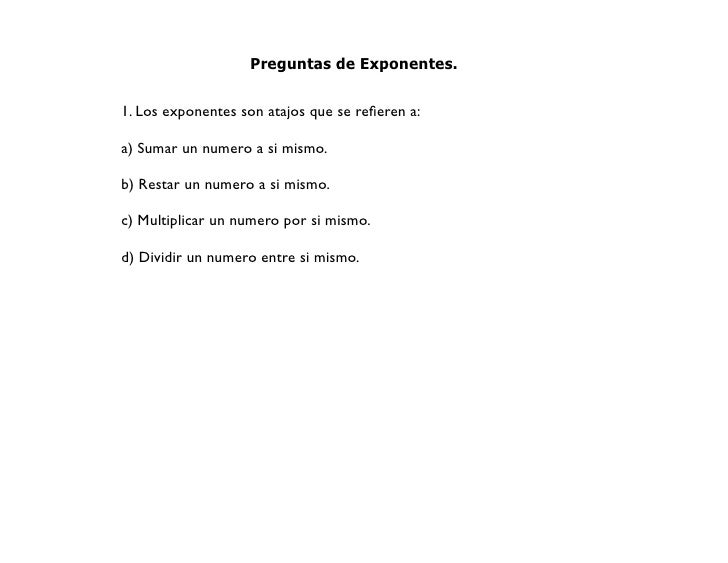Successfully reported this slideshow.Upcoming SlideShare
×

# Curso de nivelación

760 views

Published on

Estos son los ejercicios que trabajé en el curso de nivelación de julio de 2010, en el pizarrón electrónico.

Published in: Education
• Full Name
Comment goes here.

Are you sure you want to Yes No• Be the first to comment

• Be the first to like this

### Curso de nivelación

1. 1. Preguntas de Exponentes. 1. Los exponentes son atajos que se reﬁeren a: a) Sumar un numero a si mismo. b) Restar un numero a si mismo. c) Multiplicar un numero por si mismo. d) Dividir un numero entre si mismo.
2. 2. 2. Siempre que multiplicamos dos números de la misma base, podemos: a) Sumar los exponentes. b) Restar los exponentes. c) Multiplicar los exponentes. d) Dividir los exponentes.
3. 3. 3. (52)(56) se puede simpliﬁcar como sigue: 12 a) 5 . b) 58. c) 5. 26 d) 5 .
4. 4. 4. (2,87934)0: a) 0. b) 1. c) 2,879. d) Ninguno de los anteriores.
5. 5. Relacionando. (678)6 1 678 (678)0 (678)1 (6782)3
6. 6. Falso o verdadero. 1. (8/7)3 se puede simpliﬁcar como (8)3/(7)3 2. (6 + 2)2 se puede simpliﬁcar como (62 + 22)
7. 7. 34 = 81, a) ¿Que numero es la base? b) ¿Que numero es la potencia? c) ¿Que numero es el exponente?
8. 8. Evaluar, 2 3 4 5 (-1) = (-1) = (-1) = (-1) = (-1)100 = (-1)253 = 4 (-2) = 5 (-2) =
9. 9. Aplique una regla de los exponentes o reduzca términos semejantes, si es posible. a) 2x + 3x4 = 2 h) (2)(26) = 2 4 b) (2x )(3x ) = i) 35 + 35 + 35 = c) 2x3 + 3x3 = d) x2 + y2 = e) x2 + x2 = f) (x2)(x2) = g) (x2)(y3) =
10. 10. Exponente fraccionario.
11. 11. Ejercicios. Expresar con signo radical y exponentes positivos.